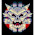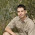## Sunday, April 1, 2018

### Optimising infinite loops with VHDL

It's been a while since I have tried to improve the CPU performance of the MEGA65, so I thought I would take a look at an optimisation I hadn't tackled yet: Accelerating infinite loops.  This sort of loop isn't normally accelerated because they occur so rarely in programs on modern computers.

However, on 8-bit systems it wasn't uncommon to have an infinite loop occupy the CPU, and the rest of the work occurring in interrupt routines.  This means that there is a surprising amount of CPU time wasted infinite loops that we can try to reduce.  We can get an idea of just how much potential benefit we can obtain by applying Amdahl's Law

Basically if 1/10th of the CPU time is spend on a particular task, this means that even if that task can be made to take no time at all, that we can only reduce the run time to 100% - 1/10, i.e., it will still take 90% of the original time.  This is where accelerating infinite loops has such great potential:  If the task would have taken infinite time, and we can reduce that to finite time, then we have gained a massive advantage.  Even better, if we can reduce the infinite time to practically zero, then we can gain an infinite speed up.  That is because, if we speed up the infinite part to take practically zero time, we have speed up the overwhelming majority of the task.  For any finite remaining run time, the ratio of speed up will be infinity / remaining run time, and since infinity divided by a finite quantity still equals infinity, the result is infinite speed up.

This all sounds great, but how can we go about achieving this in practice?  For a start, there are all sorts of infinite loops that we can have to contend with.  Again, a fortunate situation is that on 6502 systems the vast majority of infinite loops take the simple form of a JMP instruction that jumps to itself, such as:

2000 JMP \$2000

The first trick is how to efficiently detect these operations. Fortunately this is simple: If the same JMP instruction is encountered two instructions in a row, it means that the code path has become invariant, and an infinite loop will eventuate. In fact, this method is robust enough that it can be used to detect a surprisingly wide range of infinite loops. The second trick is to know how to achieve the effect of the infinite loop. Fortunately JMP instructions don't perform any other computation, or cause unpredictable changes to the processor flags, so we can, in fact, simply ignore the instruction on the second iteration, falling through to the next instruction in memory -- thus effectively executing an infinite loop in a about 12 clock cycles, i.e., 12 x 20ns = 240ns.  That is, we can execute an infinite loop in about 1/4 of a single CPU cycle on the C64, or slightly faster than a single CPU cycle on a C65.  While there is scope to further improve on this, it will be good enough for now.

So, first step is to modify the CPU to detect back-to-back jumps to the same address, and to abort the jump when this occurs.  This turned out to be trivial. Here is the complete patch:

diff --git a/src/vhdl/gs4510.vhdl b/src/vhdl/gs4510.vhdl
index e2036f7..87b4852 100644
--- a/src/vhdl/gs4510.vhdl
+++ b/src/vhdl/gs4510.vhdl
@@ -1173,6 +1173,9 @@ architecture Behavioural of gs4510 is
signal reg_math_cycle_counter_plus_one : unsigned(31 downto 0) := to_unsigned(0,32);
-- # of math cycles to trigger end of job / math interrupt
signal reg_math_cycle_compare : unsigned(31 downto 0) := to_unsigned(0,32);
+
+  signal reg_last_jump : unsigned(15 downto 0) := to_unsigned(0,16);
+  signal last_was_jump : std_logic := '0';

begin

@@ -6136,8 +6139,16 @@ begin
end if;

if reg_microcode.mcJump='1' then
-                report "Setting PC: mcJump=1";
-                reg_pc <= reg_addr;
+                if reg_last_jump /= reg_addr or last_was_jump='0' then
+                  report "Setting PC: mcJump=1";
+                  reg_pc <= reg_addr;
+                else
+                  report "Accelerating infinite loop";
+                end if;

As can be seen, all we have had to do, was to add a couple of signals to remember if the last instruction was a jump, and where the jump went. We then only take the jump if the immediately preceeding instruction was not an identical jump.

A quick test using GHDL to verify that it works under simulation was the next item on the list.  For this, I added an infinite loop at the reset entry for the MEGA65 Kickstart. This would have normally caused the MEGA65 to take an infinite amount of time to proceed with the boot process.  But now, we see the following simulation output:

@5190ns:(report note): Setting PC to \$80xx/8100 on hypervisor entry@5370ns:(report note): Setting PC: mcJump=1@5450ns:(report note): \$8100 4C 88 A1  jmp  \$A188
@5490ns:(report note): \$A188 78        sei
@5610ns:(report note): Setting PC: mcJump=1@5690ns:(report note): \$A189 4C 89 A1  jmp  \$A189
@5810ns:(report note): Accelerating infinite loop@5890ns:(report note): \$A189 4C 89 A1  jmp  \$A189

@6010ns:(report note): Setting PC in JSR/BSR (fast dispatch)
@6050ns:(report note): \$A18C 20 D8 A0  jsr  \$0104
@6090ns:(report note): \$0104 78        sei

I have highlighted the important lines above. As we can see, the infinite loop is entered at 5,610ns, and is detected at 5,810ns, and the following instruction is executed at 5,890ns.  Thus the total cost of the "infinite" loop was a mere 280ns -- a little slower than I predicted, but still quite acceptable.  It will certainly do for now, until I have time to track down where the other 40ns has gone.

#### 2 comments:

1.This is brillaint! You are a genius!
Next step, P=NP?

1.Well, you know, since we can now reduce infinite run times to small finite values, we can consider a whole pile of previously intractable problems. For example, if the decision problem, i.e., whether a program will finish or not, can be transformed to shift the infinite run time into infinite loops, then we can perhaps perform those in finite time. In that case, P=NP should also be quite straight forward. I think I might find in my diary in the morning in 364 days time to consider this problem.

Paul.